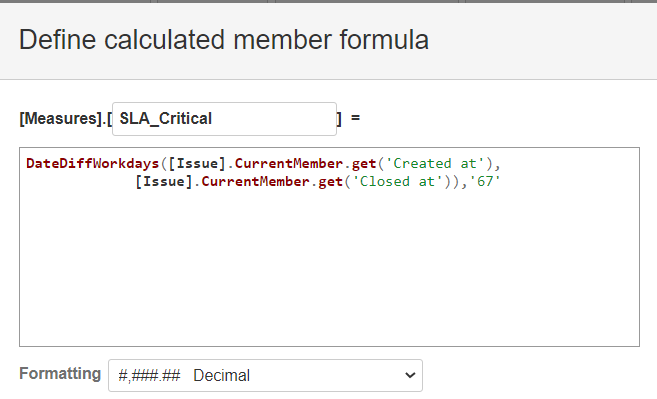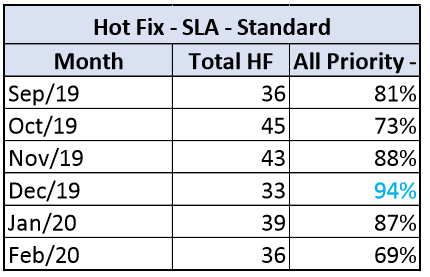# SLA report - How to create a formula to %SLA

Is there a way to create a formula to calculate like:
[Measures].[Issues resolved count],
[Measures].[SLA_Critical] < 4 (I need this to get the amount of issues that were concluded on time).

I want to create a SLA report (last image with the percentages). % of issues that were concluded on time.
Until now, I’ve only been able to create a report with a list of issues and their respectives time(create/end)Your formula calculates the difference in workdays for “Issue” dimension members at issue level as you are using two properties for issues. But the same formula would not work if you don’t use “Issue” level in the report.

To calculate results on month level (aggregated from issue results), you would need a formula that iterates through set of filtered issues (for each month) and then return the % results for SLA calculation.
Try this formula when creating a user-defined measure with % format

``````    CASE WHEN
[Measures].[Issues closed count]>0
THEN
Count(
Filter(
Descendants([Issue].CurrentHierarchyMember,[Issue].[Issue]),
DateInPeriod([Issue].Currentmember.get('Closed at'),
[Time].CurrentHierarchyMember
)
AND
DateDiffWorkdays(
[Issue].Currentmember.get('Created at'),
[Issue].Currentmember.get('Closed at'),'67'
)<4
AND
[Measures].[Issues closed]>0
)
)
/
[Measures].[Issues closed count]
END
``````

Martins / eazyBI support

1 Like

Thanks a lot!! Thats exactly what i needed